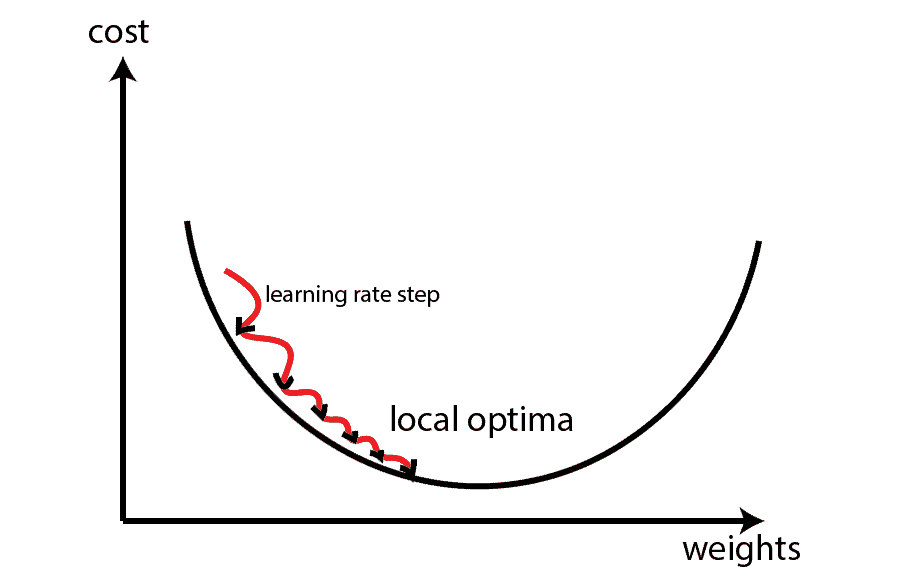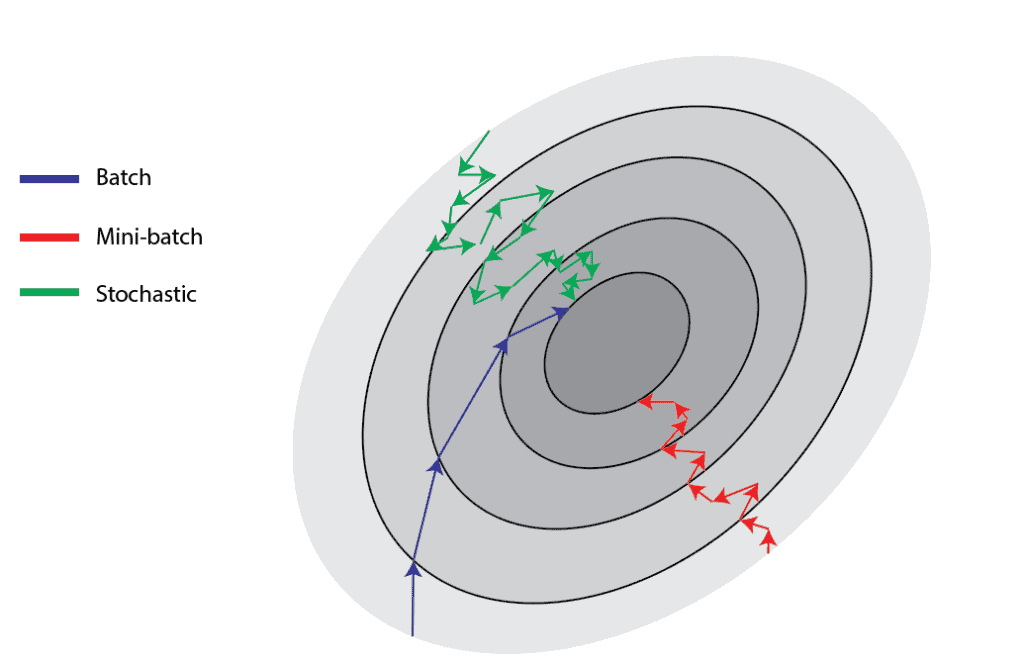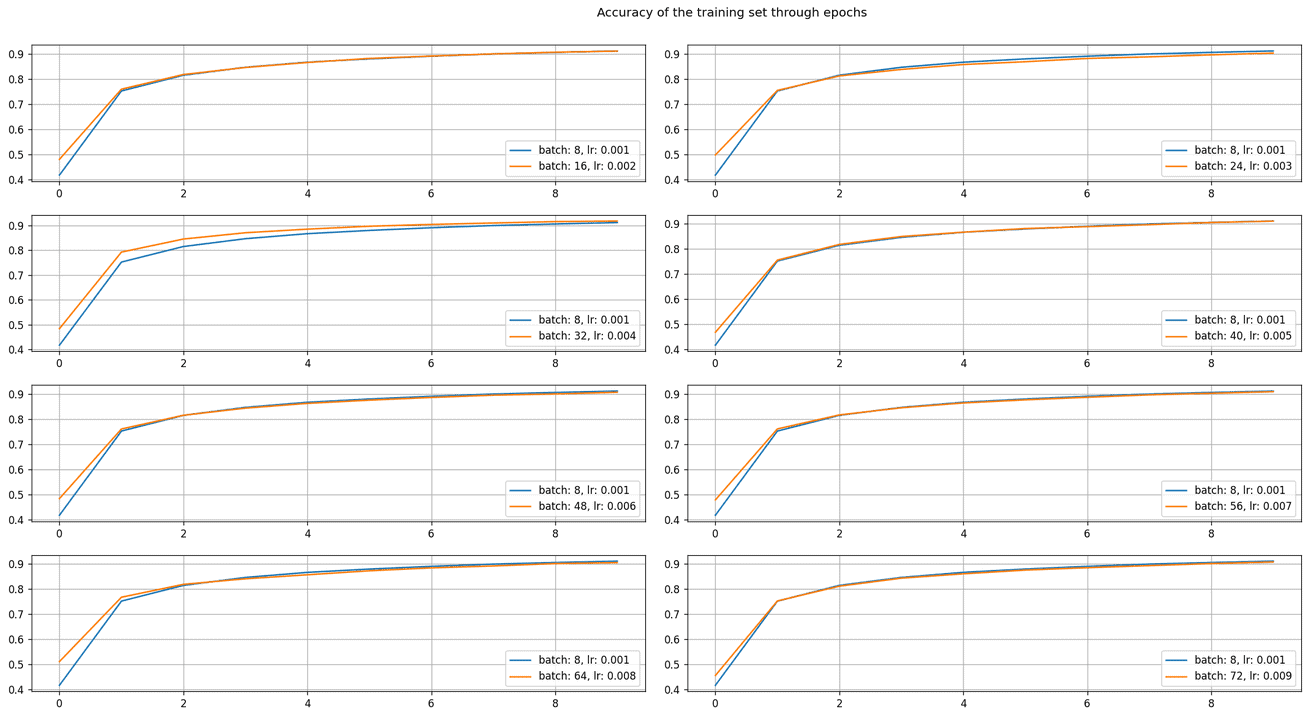## 1. Introduction

In this tutorial, we’ll discuss learning rate and batch size, two neural network hyperparameters that we need to set up before model training. We’ll introduce them both and, after that, analyze how to tune them accordingly.

Also, we’ll see how one influences another and what work has been done on this topic.

## 2. Learning Rate

Learning rate is a term that we use in machine learning and statistics. Briefly, it refers to the rate at which an algorithm converges to a solution. Learning rate is one of the most important hyperparameters for training neural networks. Thus, it’s very important to set up its value as close to the optimal as possible.

Usually, when we need to train a neural network model, we need to use some optimization techniques based on a gradient descent algorithm. After calculating the gradient of the loss function with respect to weights, that gradient has a direction to the local optima. We use a learning rate hyperparameter to tune weights towards that direction and optimize the model.

The learning rate indicates the step size that gradient descent takes towards local optima:Consequently, if the learning rate is too low, gradient descent will take more time to reach the optima. Conversely, if the learning rate is too big, the gradient descent might start to diverge, and it’ll never reach the optimal solution.

Also, the learning rate doesn’t have to have a fixed value. For example, we might define a rule that the learning rate will decrease as epochs for training increase. Besides that, some adaptive learning rate optimization methods modify the learning rate during the training. We can find more details about choosing the learning rate and gradient descent method in this article.

## 3. Batch Size

Batch size defines the number of samples we use in one epoch to train a neural network. There are three types of gradient descent in respect to the batch size:

• Batch gradient descent – uses all samples from the training set in one epoch.
• Stochastic gradient descent – uses only one random sample from the training set in one epoch.
• Mini-batch gradient descent – uses a predefined number of samples from the training set in one epoch.The mini-batch gradient descent is the most common, empirically showing the best results. For instance, let’s consider the training size of 1000 samples and the batch size of 100. A neural network will take the first 100 samples in the first epoch and do forward and backward propagation. After that, it’ll take the subsequent 100 samples in the second epoch and repeat the process.

Overall, the network will be trained for the predefined number of epochs or until the desired condition is not met.

The reason why mini-batch gradient descent is better than one single batch descent we explained in this article.

The batch size affects some indicators such as overall training time, training time per epoch, quality of the model, and similar. Usually, we chose the batch size as a power of two, in the range between 16 and 512. But generally, the size of 32 is a rule of thumb and a good initial choice.

## 4. Relation Between Learning Rate and Batch Size

The question arises is there any relationship between learning rate and batch size. Do we need to change the learning rate if we increase or decrease batch size? First of all, if we use any adaptive gradient descent optimizer, such as Adam, Adagrad, or any other, there’s no need to change the learning rate after changing batch size.

Because of that, we’ll consider that we’re talking about the classic mini-batch gradient descent method.

### 4.1. Theoretical View

There are some works done on this problem. Some authors suggest that when multiplying batch size by, we should also multiply the learning rate withto keep the variance in the gradient expectation constant. Also, more commonly, a simple linear scaling rule is used. It means that when the batch size is multiplied by, the learning rate should also be multiplied by, while other hyperparameters stay unchanged.

Namely, the authors experimented with different batch sizes and learning rates. Using the linear scaling rule, they achieved the same accuracy and matched their learning curves. They achieved this concurrence with batch sizes up toin the ImageNet experiment.

Also, they accomplished it using a gradual warmup that increments a learning rate by a constant in the first five epochs of training. This strategy prevents early overfitting by a significant learning rate.

In our example, we tried to apply the linear scaling rule. It was the experiment with MNIST data set and simple CNN with one convolutional, dropout, and fully connected layer. We compared the batch size ofand the learning rate ofwith their multiplied values, where the multiplication is done using integers fromup to. The results confirm that the learning curves are well matched.### 4.2. Practical View

The theoretical approach works well in theoretical experiments when most of the variables and data set are nearly perfect. Usually, the situation is slightly different when it comes to real-world tasks. First of all, our goal is not to match the same accuracy and learning curve using two sets of batch size and learning rate but to achieve as good as possible results.

For instance, if we increase the batch size and our accuracy increases, there’s no sense to modify the learning rate to achieve the prior results. Also, since there are many more hyperparameters to tune, we don’t know if the initial values of batch size and learning rate are optimal.

Usually, in practice, we tune these two hyperparameters with others together. Besides that, it’s common to set them up independently. For example, if the neural network trains too slow, we might increase the batch size and monitor the results’ changes. Also, we might improve the learning rate if the network converges too slow.

## 5. Conclusion

In this article, we’ve briefly described the terms batch size and learning rate. We’ve presented some theoretical background of both terms. The rule of thumb is to increase both hyperparameters from the above linearly. But also, more importantly, it’s to keep the focus on the results of the neural network and not on the ratio between batch size and learning rate.